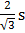# The mass and the diameter of a planet are three times the respective values for the Earth. The period of oscillation of a simple pendulum on the Earth is 2s. The period of oscillation of the same pendulum on the planet would be : a.b. 2s c.s d.s

## Question ID - 50386 :- The mass and the diameter of a planet are three times the respective values for the Earth. The period of oscillation of a simple pendulum on the Earth is 2s. The period of oscillation of the same pendulum on the planet would be : a.b. 2s c.s d.s

3537g ===   3=Also   T=== 2s

Next Question :
 Which of the following is the best conductor of electricity? (a) Aluminium (b) Copper (c) Silver (d) Gold